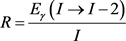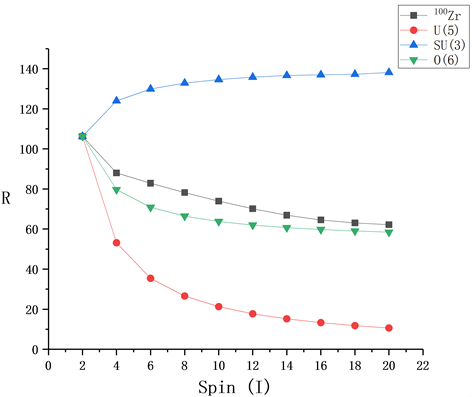# IBM模型对偶–偶核100Zr的理论研究Study of Even-Even Nuclei 100Zr by Interacting Boson Model

DOI: 10.12677/APP.2019.910049, PDF, HTML, XML, 下载: 372  浏览: 796  国家自然科学基金支持

Abstract: Even-even nuclei 100Zr were studied within the framework of the interacting boson model. The E-Gamma Over Spin (E-GOS) was drawn, and the analysis of the dynamic symmetry limit found that 100Zr is a transition nuclei from U(5) vibrational limit to SU(3) rotational limit , close to O(6) dynamic symmetry limit. At the same time, the energy spectrum of low-lying states of 100Zr was fitted, the components of the wave function were analyzed, and the B(E2) values of transitions between low-lying states of 100Zr were analyzed respectively. The results show good agreement with the available experimental data.

1. 引言

$\begin{array}{l}\text{U}\left(\text{6}\right)\supset \text{U}\left(\text{5}\right)\supset \text{O}\left(\text{5}\right)\supset \text{O}\left(\text{3}\right)\supset \text{O}\left(\text{2}\right)\\ \text{U}\left(\text{6}\right)\supset \text{SU}\left(\text{5}\right)\supset \text{O}\left(\text{3}\right)\supset \text{O}\left(\text{2}\right)\\ \text{U}\left(\text{6}\right)\supset \text{O}\left(\text{6}\right)\supset \text{O}\left(\text{5}\right)\supset \text{O}\left(\text{3}\right)\supset \text{O}\left(\text{2}\right)\end{array}$ (1)

${E}_{I}=\frac{I}{2}\hslash \omega$${E}_{\gamma }\left(I\to I-2\right)=\hslash \omega$

${E}_{I}=\frac{{\hslash }^{2}}{2J}I\left(I+1\right)$${E}_{\gamma }\left(I\to I-2\right)=\frac{{\hslash }^{2}}{2J}\left(4I-2\right)$

${E}_{I}=\frac{I\left(I+6\right)}{16}E\left({2}^{+}\right)$${E}_{\gamma }\left(I\to I-2\right)=\frac{E\left({2}^{+}\right)}{4}\left(I+2\right)$，做I-R曲线既为E-GOS曲线。

$\begin{array}{c}H=\text{EPS}{n}_{d}+\frac{1}{2}\text{ELL}\left(\stackrel{⇀}{L}\cdot \stackrel{⇀}{L}\right)+\frac{1}{2}\text{QQ}\left(\stackrel{⇀}{Q}\cdot \stackrel{⇀}{Q}\right)\\ \text{\hspace{0.17em}}\text{\hspace{0.17em}}-5\sqrt{7}\text{OCT}{\left[{\left({d}^{†}\stackrel{˜}{d}\right)}^{\left(3\right)}×{\left({d}^{†}\stackrel{˜}{d}\right)}^{\left(3\right)}\right]}_{0}^{\left(0\right)}\\ \text{\hspace{0.17em}}\text{\hspace{0.17em}}+15\text{HEX}{\left[{\left({d}^{†}\stackrel{˜}{d}\right)}^{\left(4\right)}×{\left({d}^{†}\stackrel{˜}{d}\right)}^{\left(4\right)}\right]}_{0}^{\left(0\right)}\end{array}$ (2)

$\stackrel{⇀}{L}\cdot \stackrel{⇀}{L}=-10\sqrt{3}{\left[{\left({d}^{†}\stackrel{˜}{d}\right)}^{\left(1\right)}×{\left({d}^{†}\stackrel{˜}{d}\right)}^{\left(1\right)}\right]}_{0}^{\left(0\right)}$

$\stackrel{⇀}{Q}\cdot \stackrel{⇀}{Q}=\sqrt{5}{\left[\left\{{\left({s}^{†}\stackrel{˜}{d}+{d}^{†}s\right)}^{\left(2\right)}+\frac{\text{CHQ}}{\sqrt{5}}{\left({d}^{†}\stackrel{˜}{d}\right)}^{\left(2\right)}\right\}×\left\{{\left({s}^{†}\stackrel{˜}{d}+{d}^{†}s\right)}^{\left(2\right)}+\frac{\text{CHQ}}{\sqrt{5}}{\left({d}^{†}\stackrel{˜}{d}\right)}^{\left(2\right)}\right\}\right]}_{0}^{\left(0\right)}$

2. 计算结果

2.1. 各级限值和E-GOS曲线Table 1. Experimental data and the dynamic symmetry limit of 100ZrFigure 1. Curve: the E-Gamma over spin of 100Zr

2.2. 模型参数Table 2. Hamiltonian matrix of 100Zr

2.3. 能谱结果Figure 2. Experimental energy states and theoretical energy states of 100Zr

2.4. 波函数

$|{0}_{1}^{+}〉\approx 0.5248|{s}^{10}{d}^{0}〉+0.4457|{s}^{8}{d}^{2}〉+0.0292|{s}^{6}{d}^{4}〉+0.0003|{s}^{4}{d}^{6}〉$

$\begin{array}{c}|{\Psi }_{0}^{1}〉\approx 0.724|0,0,0,0,{0}^{+}〉+0.668|2,1,0,0,{0}^{+}〉+0.171|4,2,0,0,{0}^{+}〉\\ \text{\hspace{0.17em}}\text{\hspace{0.17em}}+0.018|6,3,0,0,{0}^{+}〉+0.001|8,4,0,0,{0}^{+}〉\end{array}$

$|{0}_{2}^{+}〉\approx 0.4704|{s}^{10}{d}^{0}〉+0.4528|{s}^{8}{d}^{2}〉+0.0756|{s}^{6}{d}^{4}〉+0.0012|{s}^{4}{d}^{6}〉$

$\begin{array}{c}|{\Psi }_{0}^{2}〉\approx 0.686|0,0,0,0,{0}^{+}〉-0.673|2,1,0,0,{0}^{+}〉-0.275|4,2,0,0,{0}^{+}〉\\ \text{\hspace{0.17em}}\text{\hspace{0.17em}}-0.035|6,3,0,0,{0}^{+}〉-0.002|8,4,0,0,{0}^{+}〉\end{array}$

$|{0}_{3}^{+}〉\approx 0.8624|{s}^{6}{d}^{4}〉+0.1010|{s}^{8}{d}^{2}〉+0.0317|{s}^{4}{d}^{6}〉+0.0048|{s}^{10}{d}^{0}〉$

$\begin{array}{c}|{\Psi }_{0}^{3}〉\approx -0.069|0,0,0,0,{0}^{+}〉+0.318|2,1,0,0,{0}^{+}〉-0.929|4,2,0,0,{0}^{+}〉\\ \text{\hspace{0.17em}}\text{\hspace{0.17em}}-0.178|6,3,0,0,{0}^{+}〉-0.011|8,4,0,0,{0}^{+}〉\end{array}$

$|{2}_{1}^{+}〉\approx 0.8335|{s}^{9}{d}^{1}〉+0.1519|{s}^{7}{d}^{3}〉+0.0105|{s}^{8}{d}^{2}〉+0.0002|{s}^{6}{d}^{4}〉$

$\begin{array}{c}|{\Psi }_{2}^{1}〉\approx 0.913|1,0,0,2,{2}^{+}〉-0.103|2,0,0,2,{2}^{+}〉+0.390|3,1,0,2,{2}^{+}〉-0.015|4,1,0,2,{2}^{+}〉\\ \text{\hspace{0.17em}}\text{\hspace{0.17em}}+0.062|5,2,0,2,{2}^{+}〉-0.001|6,2,0,2,{2}^{+}〉+0.004|7,3,0,2,{2}^{+}〉\end{array}$

$\begin{array}{c}|{2}_{2}^{+}〉\approx 0.7410|{s}^{7}{d}^{3}〉+0.1203|{s}^{9}{d}^{1}〉+0.0899|{s}^{8}{d}^{2}〉+0.0434|{s}^{5}{d}^{5}〉\\ \text{\hspace{0.17em}}\text{\hspace{0.17em}}+0.0051|{s}^{6}{d}^{4}〉+0.0003|{s}^{2}{d}^{8}〉+0.0001|{s}^{3}{d}^{7}〉\end{array}$

$\begin{array}{c}|{\Psi }_{2}^{2}〉\approx -0.347|1,0,0,2,{2}^{+}〉+0.300|2,0,0,2,{2}^{+}〉+0.861|3,1,0,2,{2}^{+}〉+0.071|4,1,0,2,{2}^{+}〉\\ \text{\hspace{0.17em}}\text{\hspace{0.17em}}+0.208|5,2,0,2,{2}^{+}〉+0.007|6,2,0,2,{2}^{+}〉+0.018|7,3,0,2,{2}^{+}〉+0.001|9,4,0,2,{2}^{+}〉\end{array}$

$\begin{array}{c}|{2}_{3}^{+}〉\approx 0.8338|{s}^{8}{d}^{2}〉+0.0599|{s}^{5}{d}^{5}〉+0.0564|{s}^{7}{d}^{3}〉+0.0451|{s}^{9}{d}^{1}〉\\ \text{\hspace{0.17em}}\text{\hspace{0.17em}}+0.0040|{s}^{6}{d}^{4}〉+0.0007|{s}^{3}{d}^{7}〉\end{array}$

$\begin{array}{c}|{\Psi }_{2}^{3}〉\approx -0.212|1,0,0,2,{2}^{+}〉-0.913|2,0,0,2,{2}^{+}〉+0.238|3,1,0,2,{2}^{+}〉-0.245|4,1,0,2,{2}^{+}〉\\ \text{\hspace{0.17em}}\text{\hspace{0.17em}}+0.063|5,2,0,2,{2}^{+}〉-0.026|6,2,0,2,{2}^{+}〉-0.001|7,3,0,2,{2}^{+}〉\end{array}$

$|{2}_{4}^{+}〉\approx 0.9079|{s}^{5}{d}^{5}〉+0.0656|{s}^{8}{d}^{2}〉+0.0262|{s}^{7}{d}^{3}〉+0.0002|{s}^{9}{d}^{1}〉+0.0001|{s}^{2}{d}^{8}〉$

$\begin{array}{c}|{\Psi }_{2}^{4}〉\approx 0.014|1,0,0,2,{2}^{+}〉+0.256|2,0,0,2,{2}^{+}〉-0.002|3,1,0,2,{2}^{+}〉-0.953|4,1,0,2,{2}^{+}〉\\ \text{\hspace{0.17em}}\text{\hspace{0.17em}}-0.004|5,2,0,2,{2}^{+}〉-0.162|6,2,0,2,{2}^{+}〉-0.009|7,3,0,2,{2}^{+}〉\end{array}$

$|{4}_{1}^{+}〉\approx 0.9348|{s}^{8}{d}^{2}〉+0.0646|{s}^{6}{d}^{4}〉+0.0007|{s}^{4}{d}^{4}〉$

$|{\Psi }_{4}^{1}〉\approx 0.967|2,0,0,4,{4}^{+}〉+0.254|4,1,0,4,{4}^{+}〉+0.027|6,2,0,4,{4}^{+}〉+0.001|8,3,0,4,{4}^{+}〉$

$|{4}_{2}^{+}〉\approx 0.9087|{s}^{6}{d}^{4}〉+0.0650|{s}^{8}{d}^{2}〉+0.0263|{s}^{4}{d}^{6}〉+0.0001|{s}^{2}{d}^{8}〉$

$|{\Psi }_{4}^{2}〉\approx -0.225|2,0,0,4,{4}^{+}〉+0.953|4,1,0,4,{4}^{+}〉+0.162|6,2,0,4,{4}^{+}〉+0.009|8,3,0,4,{4}^{+}〉$

$|{4}_{3}^{+}〉\approx 0.9689|{s}^{7}{d}^{3}〉+0.0309|{s}^{5}{d}^{5}〉+0.0002|{s}^{3}{d}^{7}〉$

$|{\Psi }_{4}^{3}〉\approx 0.984|3,0,0,4,{4}^{+}〉+0.176|5,1,0,4,{4}^{+}〉+0.013|7,2,0,4,{4}^{+}〉$

$|{4}_{4}^{+}〉\approx 0.9549|{s}^{5}{d}^{5}〉+0.0310|{s}^{7}{d}^{3}〉+0.0140|{s}^{3}{d}^{7}〉$

$|{\Psi }_{4}^{4}〉\approx -0.176|3,0,0,4,{4}^{+}〉+0.977|5,1,0,4,{4}^{+}〉+0.118|7,2,0,4,{4}^{+}〉+0.004|9,3,0,4,{4}^{+}〉$

2.5. 电磁跃迁Table 3. The B(E2) of electromagnetic transitions of 100Zr

3. 结论

  Iachello, F. and Arima, A. (1987) The Interacting Boson Model. Cambridge University Press, Cambridge. https://doi.org/10.1017/CBO9780511895517  Arima, A. and Iachello, F. (1976) Interacting Boson Model of Collective States I. The Vibrational Limit. Annals of Physics, 99, 253-317. https://doi.org/10.1016/0003-4916(76)90097-X  Arima, A. and Iachello, F. (1978) Interacting Boson Model Of Collective Nuclear States II. The Rotational Limit. Annals of Physics, 111, 201-238.https://doi.org/10.1016/0003-4916(78)90228-2  Arima, A. and Iachello, F. (1979) Interacting Boson Model of Collective Nuclear States IV. The O(6) Limit. Annals of Physics, 123, 468-492.https://doi.org/10.1016/0003-4916(79)90347-6  Pan, F., Draayer, J.P. and Luo, Y.A. (2003) A Close Look at U(5)↔SU(3) Transitional Patterns in the Interacting Boson Model. Physics Letters B, 576, 297-302.https://doi.org/10.1016/j.physletb.2003.09.098  Liu, Y.X., Mu, L.Z. and Wei, H.Q. (2006) Approach to the Rotation Driven Vibrational to Axially Rotational Shape Phase Transition along the Yrast Line of a Nucleus. Physics Letters B, 633, 49-53. https://doi.org/10.1016/j.physletb.2005.11.018  Mu, L.Z. and Liu, Y.X. (2005 ) Rotation Driven Shape-Phase Transition of the Yrast Nuclear States with O(6) Symmetry in the Interacting Boson Model. Chinese Physics Letters, 22, 1354-1357. https://doi.org/10.1088/0256-307X/22/6/016  Zhang, J.F., Lv, L.J. and Bai, H.B. (2007) Critical Behavior in Nuclear Structure from Spherical to Axially Symmetric Deformed Shape in IBM. Chinese Physics, 16, 1841-1846. https://doi.org/10.1088/1009-1963/16/7/022  Liu, Y.X., Sun, Y., Zhou, X.H., et al. (2011) A Systematical Study of Neutron-Rich Zr Isotopes by the Projected Shell Model. Nuclear Physics A, 858, 11-31. https://doi.org/10.1016/j.nuclphysa.2011.03.010